# can't get coordinates straight

## Recommended PostsI have set in autocad the correct angle settings, have been applying 20 different functions to obtain the correct angle (Y axis) between two coordinates, but I keep getting strange angles back from this routine. Either the correct angle is difference with 90, or 450. Any idea why? I have gone through most of math forums on the internet. I need the angle correctly in order to scale a polygon.

thanks.

Public Function GiveAngle(DegRad As Boolean, x1 As Double, y1 As Double, x2 As Double, y2 As Double) As Double

Dim Xdist, Ydist As Double

Dim ATAN3 As Double

Dim PI As Double

PI = 3.14159265358979

Xdist = (x2 - x1)

Ydist = (y2 - y1)

If Abs(Ydist) > Abs(Xdist) Then

If Ydist > 0 Then

ATAN3 = Math.Atn((x2 - x1) / (y1 - y2))

Else

ATAN3 = Math.Atn((x2 - x1) / (y1 - y2)) + PI

End If

Else

If Xdist > 0 Then

ATAN3 = 0.5 * PI - Atn((x2 - x1) / (y1 - y2))

Else

ATAN3 = -0.5 * PI - Atn((x2 - x1) / (y1 - y2))

End If

End If

GiveAngle = ATAN3 * 180 / PI

End Function

##### Share on other sites... to obtain the correct angle (Y axis) between two coordinates

The Y angle between two coordinates is simply the angle between same + (0.5 * Pi), no?

```       public double GiveAngle(Point2d pt1, Point2d pt2)        // C# code
{
return pt1.GetVectorTo(pt2).Angle + (0.5 * Math.PI);
}
```

 - Separately, please use [code ] Tags.

##### Share on other sitesFirst of all read up on how you post your code or you will get a moderator onto you.

Your logic is a bit off and will not deliver correct answers. You need to consider which quadrant you are in and the special cases when the angle is due E, N , W or S. The resulting code can get a little complicated so I would recommend another approach. You have the starting point and end point coordinates, so use them to draw a temporary line, interrogate the line to get its angle and delete the temporary line.

This code should work for you if you adapt it to your situation:

```   Dim basePnt As Variant
Dim returnPnt As Variant
Dim Bearing As Double
Dim AngleXY As Double
Dim PI As Double

' Set the value for PI
PI = Math.Atn(1#) * 4

' select points on screen
basePnt = ThisDrawing.Utility.GetPoint(, vbCrLf & "Select first point...")
returnPnt = ThisDrawing.Utility.GetPoint(basePnt, "Select second point...")

' Create a line from the base point and the last point entered

' Get angle of line (rads) and convert it to degrees
AngleXY = DistLine.Angle
Bearing = AngleXY * 180# / PI

' Format the result
Bearing = Format(Bearing, "##0.00000")

' Display result in a message box
MsgBox "Angle from system zero =   " & Bearing & "°" _
, vbOKOnly, "3D-Angles"

' Delete temporary line
DistLine.Delete

```

I hope that helps.

@ BlackBox:

Nice neat and concise code. Unfortunately it doesn't work in VBA, as far as I am aware.

##### Share on other sitesFirstly, I'm having a _really_ rough Monday morning, even for a Monday morning... So kindly educate me, where I must be overlooking the obvious....

You need to consider which quadrant you are in and the special cases when the angle is due E, N , W or S... ... You have the starting point and end point coordinates, so use them to draw a temporary line, interrogate the line to get its angle and delete the temporary line.

Given the OP's request to derive Y angle between two coordinates, I am not sure I understand the relevance of quadrant (tabling the line aspect for now)... The X angle is always the angle between start point and end point, thus Y angle will always be X angle + (0.5 * Pi), no?

Cheers

##### Share on other sitesFirstly, I'm having a _really_ rough Monday morning, even for a Monday morning... So kindly educate me, where I must be overlooking the obvious....

Given the OP's request to derive Y angle between two coordinates, I am not sure I understand the relevance of quadrant (tabling the line aspect for now)... The X angle is always the angle between start point and end point, thus Y angle will always be X angle + (0.5 * Pi), no?

Cheers

Sorry to hear that your start to the week is not going too well, my Monday is over now and I'm off to enjoy a nice red wine with my little lady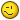.

You could be right with your assumption that it is just a simple matter of finding the acute angle to the X axis and if that is so my solution is not what the OP is looking for. But this code will give him the answer that he's after:

```
Xdist = Abs(x2 - x1): Ydist = Abs(y2 - y1)
ATAN3 = Math.Atn(Ydist / Xdist)
dAngle = ATAN3 * 180 / PI
```

##### Share on other sitesSorry to hear that your start to the week is not going too well, my Monday is over now and I'm off to enjoy a nice red wine with my little lady.

Me too!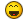It has nothing to do with CAD either; I'll reserve comment as it is off topic. Nonetheless, thanks for the well wishes, and I hope you and the captivating Mrs. Tyke enjoy your vino.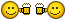You could be right with your assumption that it is just a simple matter of finding the acute angle to the X axis and if that is so my solution is not what the OP is looking for. But this code will give him the answer that he's after:

```
Xdist = Abs(x2 - x1): Ydist = Abs(y2 - y1)
ATAN3 = Math.Atn(Ydist / Xdist)
dAngle = ATAN3 * 180 / PI
```

That is a nice demonstration of Pythagorean theorem, but is essentially a verbose version of the GetVectorTo() Method, no?

##### Share on other sitesI just saw this:

@ BlackBox:

Nice neat and concise code. Unfortunately it doesn't work in VBA, as far as I am aware.

Ohhhh... This is VBA? I thought the OP posted VB.NET (hence the C# reply)!These languages really need to be split up into their own forums for clarity of those seeking help, and those who might offer it.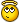Cheers

##### Share on other sitesIf VBA, why not use:

```ThisDrawing.Utility.AngleFromXAxis(pt1, pt2)
```

 - ... + (0.5 * Pi), of course.

##### Share on other sitesThat is a nice demonstration of Pythagorean theorem, but is essentially a verbose version of the GetVectorTo() Method, no?

Yes it is verbose, but unfortunately VBA is not as concise as VLISP or C#. But on the other hand, much more concise than the OP's original code.Let's wait and see what the OP says, perhaps we have both missed his point.

##### Share on other sitesIf VBA, why not use:

```ThisDrawing.Utility.AngleFromXAxis(pt1, pt2)
```

 - ... + (0.5 * Pi), of course.

Why not indeed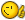, but would you need to have:

`(0.5 * Pi) - ThisDrawing.Utility.AngleFromXAxis(pt1, pt2)`

ie 90° minus angle from X-Axis?

##### Share on other sitesYes it is verbose, but unfortunately VBA is not as concise as VLISP or C#. But on the other hand, much more concise than the OP's original code.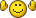Let's wait and see what the OP says, perhaps we have both missed his point.

Agreed.

Why not indeed, but would you need to have:

```(0.5 * Pi) - ThisDrawing.Utility.AngleFromXAxis(pt1, pt2)
```

ie 90° minus angle from X-Axis?

If XAngle == 45 degrees (1/4 Pi), then subtracting it from 1/2 Pi, yields 1/4 Pi... However, adding 1/2 Pi, yields 3/4 Pi.##### Share on other sites:If XAngle == 45 degrees (1/4 Pi), then subtracting it from 1/2 Pi, yields 1/4 Pi... However, adding 1/2 Pi, yields 3/4 Pi.And the maximum angle from the Y-axis is 1/2 Pi (90°).

All we need now is a response from the OP.

##### Share on other sitesAnd the maximum angle from the Y-axis is 1/2 Pi (90°).

We're deriving YAngle from XAngle, and not deriving XAngle from YAngle (as there is no built-in Method for YAngle), thus one must add 1/2 Pi to XAngle.

##### Share on other sitesWe're deriving YAngle from XAngle, and not deriving XAngle from YAngle (as there is no built-in Method for YAngle), thus one must add 1/2 Pi to XAngle.

Absolutely correctBut am I missing something here, if we are deriving the angle of the line from the Y-axis it can not be greater than 1/2 Pi (90°) and by adding 1/2 Pi to the XAngle will always give a value of greater than 90°, because 1/2 Pi is 90°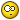##### Share on other sitesBut am I missing something here, if we are deriving the angle of the line from the Y-axis it can not be greater than 1/2 Pi (90°) and by adding 1/2 Pi to the XAngle will always give a value of greater than 90°, because 1/2 Pi is 90°As I understand it, the OP merely requested YAngle, given the equivalent of Point2d (X, Y) for start and end points, thus simply calculating the (X)Angle of start-->end allows us to add 1/2 Pi to yield YAngle, regardless of its orientation, or quadrant.

Generally, AutoCAD will automagically subtract 2*Pi in the event the returned Vector2D is greater at input (i.e., at Command Line, etc.), but even that could be a simple evaluation within the Method/Function being coded here.

Visual LISP Example:

```(vla-put-rotation <BlockReferenceObject> (+ (* 0.5 pi) (* 2 pi)))
```

Now, should the OP be intending for the resultant YAngle being calculated to always be 'plan readable' that has not yet been requested, so based on the information given thus far, the aforementioned is the best, and simplest I can come up with, regardless of API being used.

Cheers

## Join the conversation

You can post now and register later. If you have an account, sign in now to post with your account.
Note: Your post will require moderator approval before it will be visible.×   Pasted as rich text.   Paste as plain text instead

Only 75 emoji are allowed.